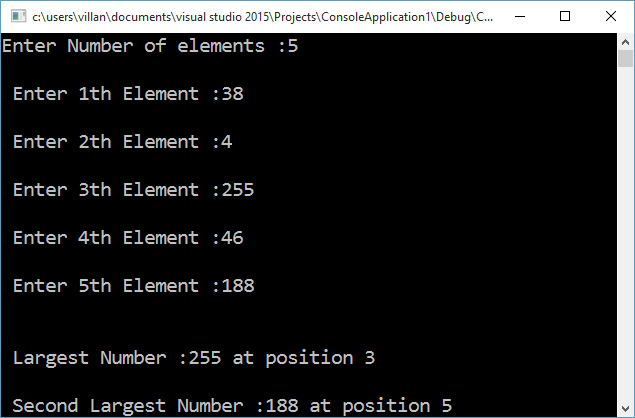# C++ program to find the largest and second largest number present in an arrayC++ program to find the largest and second-largest numbers present in an array along with their location index on the array. This program first find the largest number by going through all the numbers in the array. It stores the index of the largest number on the variable pos1 and largest number is the largest variable. A second for loop is applied, which also check for the largest number. When it hits on the largest found by the first loop, it is bypassed using a continue statement and hence will get the second-largest number.

```#include <iostream>
#include <conio.h>
using namespace std;

void main()
{
int a, i, largest = 0, second_largest = 0, pos1, pos2;
int n;
cout << "Enter Number of elements :";
cin >> n;
for (i = 0; i < n; ++i)
{
cout << "n Enter " << (i + 1) << "th Element :";
cin >> a[i];
}
//Finding Largest
for (i = 0; i < 10; ++i)
{
if (a[i] > largest)
{
largest = a[i];
pos1 = i;
}
}
//finding second largset
for (i = 0; i < 10; ++i)
{
if (a[i] > second_largest)
{
if (a[i] == largest)
continue;	//Ignoring largest in order to get second largest
second_largest = a[i];
pos2 = i;
}
}
cout << "nn Largest Number :" << largest << " at position " << (pos1 + 1);
cout << "nn Second Largest Number :" << second_largest << " at position " << (pos2 + 1);

getch();
return;
}
```

*Compiled using Visual StudioMuhammed Afsal Villan is an experienced full-stack developer, specialized in desktop and mobile application development. He also regularly publishes quality tutorials on his YouTube channel named 'Genuine Coder'. He likes to contribute to open-source projects and is always enthusiastic about new technologies.